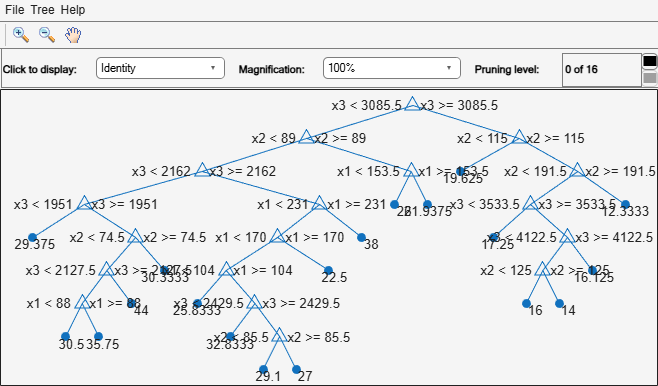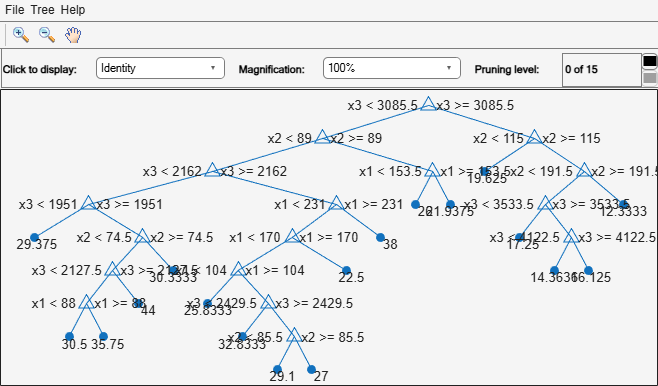# loss

Regression error

## Syntax

``L = loss(tree,tbl,ResponseVarName)``
``L = loss(tree,x,y)``
``L = loss(___,Name,Value)``
``````[L,se,NLeaf,bestlevel] = loss(___)``````

## Description

````L = loss(tree,tbl,ResponseVarName)` returns the mean squared error between the predictions of `tree` to the data in `tbl`, compared to the true responses `tbl.ResponseVarName`.```
````L = loss(tree,x,y)` returns the mean squared error between the predictions of `tree` to the data in `x`, compared to the true responses `y`.```
````L = loss(___,Name,Value)` computes the error in prediction with additional options specified by one or more `Name,Value` pair arguments, using any of the previous syntaxes.```
``````[L,se,NLeaf,bestlevel] = loss(___)``` also returns the standard error of the loss (`se`), the number of leaves (terminal nodes) in the tree (`NLeaf`), and the optimal pruning level for `tree` (`bestlevel`).```

## Input Arguments

expand all

Trained regression tree, specified as a `RegressionTree` object constructed by `fitrtree` or a `CompactRegressionTree` object constructed by `compact`.

Predictor values, specified as matrix of floating-point values. Each column of `x` represents one variable, and each row represents one observation.

Data Types: `single` | `double`

Response variable name, specified as the name of a variable in `tbl`.

You must specify `ResponseVarName` as a character vector or string scalar. For example, if the response variable `y` is stored as `tbl.y`, then specify `ResponseVarName` as `'y'`. Otherwise, the software treats all columns of `tbl`, including `y`, as predictors when training the model.

Data Types: `char` | `string`

Response data, specified as a numeric column vector with the same number of rows as `x`. Each entry in `y` is the response to the data in the corresponding row of `x`.

Data Types: `single` | `double`

### Name-Value Pair Arguments

Specify optional comma-separated pairs of `Name,Value` arguments. `Name` is the argument name and `Value` is the corresponding value. `Name` must appear inside quotes. You can specify several name and value pair arguments in any order as `Name1,Value1,...,NameN,ValueN`.

Loss function, specified as the comma-separated pair consisting of `'LossFun'` and a function handle for loss, or `'mse'` representing mean-squared error. If you pass a function handle `fun`, `loss` calls `fun` as:

`fun(Y,Yfit,W)`
• `Y` is the vector of true responses.

• `Yfit` is the vector of predicted responses.

• `W` is the observation weights. If you pass `W`, the elements are normalized to sum to `1`.

All the vectors have the same number of rows as `Y`.

Example: `'LossFun','mse'`

Data Types: `function_handle` | `char` | `string`

Pruning level, specified as the comma-separated pair consisting of `'Subtrees'` and a vector of nonnegative integers in ascending order or `'all'`.

If you specify a vector, then all elements must be at least `0` and at most `max(tree.PruneList)`. `0` indicates the full, unpruned tree and `max(tree.PruneList)` indicates the completely pruned tree (i.e., just the root node).

If you specify `'all'`, then `loss` operates on all subtrees (i.e., the entire pruning sequence). This specification is equivalent to using `0:max(tree.PruneList)`.

`loss` prunes `tree` to each level indicated in `Subtrees`, and then estimates the corresponding output arguments. The size of `Subtrees` determines the size of some output arguments.

To invoke `Subtrees`, the properties `PruneList` and `PruneAlpha` of `tree` must be nonempty. In other words, grow `tree` by setting `'Prune','on'`, or by pruning `tree` using `prune`.

Example: `'Subtrees','all'`

Data Types: `single` | `double` | `char` | `string`

Tree size, specified as the comma-separated pair consisting of `'TreeSize'` and one of the following:

• `'se'``loss` returns `bestlevel` that corresponds to the smallest tree whose mean squared error (MSE) is within one standard error of the minimum MSE.

• `'min'``loss` returns `bestlevel` that corresponds to the minimal MSE tree.

Example: `'TreeSize','min'`

Observation weights, specified as the comma-separated pair consisting of `'Weights'` and a vector of scalar values. The software weights the observations in each row of `x` or `tbl` with the corresponding value in `Weights`. The size of `Weights` must equal the number of rows in `x` or `tbl`.

If you specify the input data as a table `tbl`, then `Weights` can be the name of a variable in `tbl` that contains a numeric vector. In this case, you must specify `Weights` as a variable name. For example, if weights vector `W` is stored as `tbl.W`, then specify `Weights` as `'W'`. Otherwise, the software treats all columns of `tbl`, including `W`, as predictors when training the model.

Data Types: `single` | `double` | `char` | `string`

## Output Arguments

expand all

Classification error, returned as a vector the length of `Subtrees`. The error for each tree is the mean squared error, weighted with `Weights`. If you include `LossFun`, `L` reflects the loss calculated with `LossFun`.

Standard error of loss, returned as a vector the length of `Subtrees`.

Number of leaves (terminal nodes) in the pruned subtrees, returned as a vector the length of `Subtrees`.

Best pruning level as defined in the `TreeSize` name-value pair, returned as a scalar whose value depends on `TreeSize`:

• `TreeSize` = `'se'``loss` returns the highest pruning level with loss within one standard deviation of the minimum (`L`+`se`, where `L` and `se` relate to the smallest value in `Subtrees`).

• `TreeSize` = `'min'``loss` returns the element of `Subtrees` with smallest loss, usually the smallest element of `Subtrees`.

## Examples

expand all

Load the `carsmall` data set. Consider `Displacement`, `Horsepower`, and `Weight` as predictors of the response `MPG`.

```load carsmall X = [Displacement Horsepower Weight];```

Grow a regression tree using all observations.

`tree = fitrtree(X,MPG);`

Estimate the in-sample MSE.

`L = loss(tree,X,MPG)`
```L = 4.8952 ```

Load the `carsmall` data set. Consider `Displacement`, `Horsepower`, and `Weight` as predictors of the response `MPG`.

```load carsmall X = [Displacement Horsepower Weight];```

Grow a regression tree using all observations.

`Mdl = fitrtree(X,MPG);`

View the regression tree.

`view(Mdl,'Mode','graph');`Find the best pruning level that yields the optimal in-sample loss.

```[L,se,NLeaf,bestLevel] = loss(Mdl,X,MPG,'Subtrees','all'); bestLevel```
```bestLevel = 1 ```

The best pruning level is level 1.

Prune the tree to level 1.

```pruneMdl = prune(Mdl,'Level',bestLevel); view(pruneMdl,'Mode','graph');```Unpruned decision trees tend to overfit. One way to balance model complexity and out-of-sample performance is to prune a tree (or restrict its growth) so that in-sample and out-of-sample performance are satisfactory.

Load the `carsmall` data set. Consider `Displacement`, `Horsepower`, and `Weight` as predictors of the response `MPG`.

```load carsmall X = [Displacement Horsepower Weight]; Y = MPG;```

Partition the data into training (50%) and validation (50%) sets.

```n = size(X,1); rng(1) % For reproducibility idxTrn = false(n,1); idxTrn(randsample(n,round(0.5*n))) = true; % Training set logical indices idxVal = idxTrn == false; % Validation set logical indices```

Grow a regression tree using the training set.

`Mdl = fitrtree(X(idxTrn,:),Y(idxTrn));`

View the regression tree.

`view(Mdl,'Mode','graph');`The regression tree has seven pruning levels. Level 0 is the full, unpruned tree (as displayed). Level 7 is just the root node (i.e., no splits).

Examine the training sample MSE for each subtree (or pruning level) excluding the highest level.

```m = max(Mdl.PruneList) - 1; trnLoss = resubLoss(Mdl,'SubTrees',0:m)```
```trnLoss = 7×1 5.9789 6.2768 6.8316 7.5209 8.3951 10.7452 14.8445 ```
• The MSE for the full, unpruned tree is about 6 units.

• The MSE for the tree pruned to level 1 is about 6.3 units.

• The MSE for the tree pruned to level 6 (i.e., a stump) is about 14.8 units.

Examine the validation sample MSE at each level excluding the highest level.

`valLoss = loss(Mdl,X(idxVal,:),Y(idxVal),'SubTrees',0:m)`
```valLoss = 7×1 32.1205 31.5035 32.0541 30.8183 26.3535 30.0137 38.4695 ```
• The MSE for the full, unpruned tree (level 0) is about 32.1 units.

• The MSE for the tree pruned to level 4 is about 26.4 units.

• The MSE for the tree pruned to level 5 is about 30.0 units.

• The MSE for the tree pruned to level 6 (i.e., a stump) is about 38.5 units.

To balance model complexity and out-of-sample performance, consider pruning `Mdl` to level 4.

```pruneMdl = prune(Mdl,'Level',4); view(pruneMdl,'Mode','graph')```